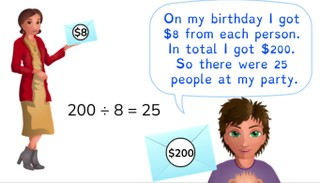Mental math- division to 1,000

# Mental math- division to 1,000

Students learn division using mental math with numbers to 1,000.

No account needed.8,000 schools use Gynzy92,000 teachers use Gynzy1,600,000 students use Gynzy

## General

The students learn division using mental math with numbers to 1,000.

## Standards

CCSS.Math.Content.5.NBT.B.5

## Learning objective

Students will be able to divide to 1,000 using mental math.

## Introduction

Have the students solve the problems within the time given by the timer.

## Instruction

Explain that with mental math division you can solve problems in your head in a quick way. Emphasize that you aren't going to use looseleaf or graphing paper for this lesson. Show that you can solve problems in a variety of ways. So you look at the problem to see what the easiest way is for you to solve the problem. One way to do this is by splitting. Use the example of 830 ÷ 10. First you solve 800 ÷ 10, then 30 ÷ 10 and then you add the answers together. Another method is doubling a problem. When you double the problem 250 ÷ 50, it becomes 500 ÷ 100. This problem is easier to solve and the answer will be the same. Next you show how you can solve a problem using halving. When you halve the problem 160 ÷ 8 it becomes the easier problem 80 ÷ 4. The answers are the same here as well. Finally, discuss the zeroes rule as a simple way to solve division problems. Solve 80 ÷ 4 using the helping problem 8 ÷ 4 = 2, and then add the 0 back on. Then the answer is 20. Discuss the problem 600 ÷ 4. Ask what method the students would use to solve this problem using mental math. Show the methods that the children use by removing the covers. Repeat this with the problem 920 ÷ 10. The students can solve the following three problems on their own. Show the story problem. Walk through the steps of solving a story problem together.

Check whether the students can divide using mental math, by asking the following question:
- How would you solve the problem 720 ÷ 80 using mental math?

## Quiz

The students test their understanding of division through mental math by completing ten exercises. Each exercise is a division problem which should be solved mentally. Some of the exercises are story problems.

## Closing

Discuss once again the importance of division using mental math. As a closing activity, have the students solve the problems. Discuss with the students which method they used for each problem, and why.

## Teaching tips

Have students that have difficulty with division using mental math, first practice with the division tables.

### The online teaching platform for interactive whiteboards and displays in schools

• Save time building lessons

• Manage the classroom more efficiently

• Increase student engagement